﻿ DPSFHelper.RandomPointOnCircleVector3 Method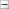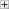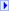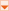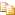Collapse AllExpand AllCode: All Code: Multiple Code: C# Code: Visual Basic Code: Visual C++

[TCheckBoxFormControlObj]C#

[TCheckBoxFormControlObj]Visual Basic

[TCheckBoxFormControlObj]
Visual C++
 DPSF API Documentation DPSFHelper . . :: ..RandomPointOnCircleVector3 MethodReturns a random point on a circle with a radius of one, on the X-Y axis plane (Z value of zero). To use a different radius simply multiply the returned value by the desired radius value.

Namespace:

Assembly: DPSF (in DPSF.dll) Version: 2.5.0.0Syntax

 C# public static Vector3 RandomPointOnCircleVector3()
 Visual Basic Public Shared Function RandomPointOnCircleVector3 As Vector3
 Visual C++ public: static Vector3 RandomPointOnCircleVector3()

Return Value

Type: Vector3

Returns a random 3D position on a circle, with a Z value of zeroSee Also

DPSFHelper Class

DPSFHelper Members

DPSF NamespaceSend comments on this topic to